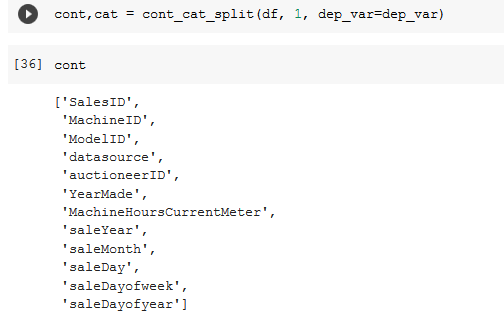# Selecting categorical and continuous variables

I am struggling with understanding what constitutes a categorical versus continuous variable.

In Notebook 9 (tabular) we are introduced to `cont_cat_split()` to automatically separate continuous from categorical variables. For tractor bluebook, when I call list of continuous variables, I get the following:If I look at the ‘auctioneerID’ column, there are 31 unique auctioneers, and 412 698 entries. I expect that this is just a mischaraterization by the helper function, i.e. it should be categorical, not continuous. But how do we decide?

For example, is ‘saleDay’ continuous? There is some ordinality to days of the week but they aren’t continuous like height or temperature. Same with many of the other continuous variables here.

Meanwhile, ‘Blade_Width’ has a range from <12’ to 16’ (with many values missing). I’d argue that it’s categorical (as the helper function specifies) but is it more categorical than saleMonth, which is taken as a continuous variable?

So my questions are:

1. How you decide what to call categorical and what to call continuous when they have numbers that are ordinal?
2. How is the helper function deciding?
3. What is the role of the parameter `max_card` and why was `max_card=1` chosen?
4. How do I override if I want to change what what the helper function specifies as a categorical or continuous variable?
2 Likes

I bucket categorical and continuous based on the following criteria:

1. Is it sets? Such as Jack/Queen/King? Then it’s categorical
2. Do my numbers carry specific meaning? Such as 1st, 2nd, 3rd? Then it’s categorical
3. Otherwise, it’s a continuous variable

To answer it even more, here is an excerpt from `fastbook`, chapter 9 on tabular:

Continuous and Categorical Variables: Continuous variables are numerical data, such as “age,” that can be directly fed to the model, since you can add and multiply them directly. Categorical variables contain a number of discrete levels, such as “movie ID,” for which addition and multiplication don’t have meaning (even if they’re stored as numbers).

Take a look at `cont_cat_split??`

`max_card` dictates what the maximum cardinality is (number of categories/different values per variable). So anything with more than one unique value in this case will be thrown into a continuous variable.

Just declare your cats and conts yourself. It’s just a helper function, you certainly don’t need to use it. Just list out `cat_names = [blah]`, `cont_names = [blah]`

Here you are dealing with time series which is different from the bulldozer problem. I would recommend watching last years lesson on Rossmann as it covers how time series data is more categorical and how you can feature engineer it. Those notebooks (done in the new fastai) can be found here:

In such a case you should opt for declaring your variables separately rather than the helper function

5 Likes

I think `max_card=1` (for the bulldozer dataset) is a typo in the book. Any categorical variable will have cardinality greater than 1. Otherwise, what’s the point of the categorical variable?